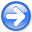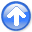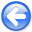Tomographic full waveform inversion and linear modeling of multiple scatteringNext: Gradient computation with TFWI Up: Biondi: TFWI and multiple Previous: Multiple-scattering modeling

# Tomographic Full Waveform Inversion (TFWI)

We can rewrite equations 5-6 by performing the following substitution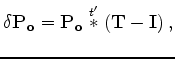(12)

and consequently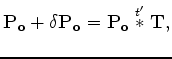(13)

whereis a convolutional operator in time that may vary both in space and time;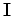is the identity operator. For example, when the perturbed wavefield is a time-shifted version of the background wavefield, the operatoris a shifted delta function. With this substitution equation 6 can be rewritten as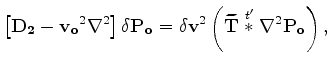(14)

where the substitution ofwithtakes into account of the Laplacian.

If we define an velocity model extended in time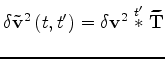, we can rewrite equation 14 as(15)

The estimation of an extended velocity as a function of both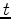and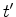, and for each seismic experiment (e.g. shot), can be unpractical. We can approximate equation 15 by making the velocity dependent only from the convolutional time lag; that is,and the same for each seismic experiment. The approximation of equation 15 can be written as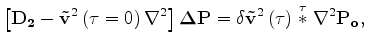(16)

where the change of notation from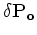to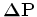indicates that the scattered wavefieldis now an approximation of the true multiple-scattered wavefield.

Formally solving equation 16 we obtain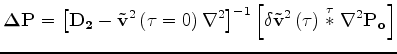(17)

that is a linear relationship between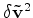anddefined by the linear operator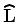such as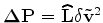.

If we define the total wavefield to be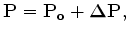(18)

and the extended non-linear modeling operator as(19)

the objective function(20)

has the same local minima of the original FWI objective function, but it also provides smooth descending paths to the global minimum in the additional dimensions. The problem is now under constrained because many solutions fit the data equally well. Among all these possible solutions we are interested in the solutions for which the extended velocity model is as focused as possible around the zero time lag of the model.

To converge towards a desirable solution we can add an additional term to the objective function that penalizes extended velocity model with significant energy at non-zero time lag; that is,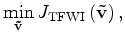(21)

with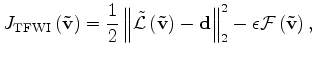(22)

whereis an operator that measure the focusing of the model at zero time lag. A straightforward example of such operator is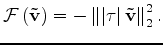(23)

SubsectionsTomographic full waveform inversion and linear modeling of multiple scatteringNext: Gradient computation with TFWI Up: Biondi: TFWI and multiple Previous: Multiple-scattering modeling

2012-10-29